# Compelling Advancements of the RNN (Seq2Seq Learning and Structured Attention Networks)

Prior knowledge recommended: NLP, RNNs, LSTMs, attention.

# Introduction

RNN-based architectures are relevant and popular choices for a large variety of machine learning tasks. In particular, tasks like speech recognition, natural language processing, video processing, anomaly detection, time-series prediction, and others involving sequential data require a nuanced modeling approach that calls for remembering previous states and synthesizing context surrounding an element’s position in a sequence. This cutting-edge research field has birthed a plethora of compelling advancements and in this blog post I will go over 2 papers encompassing several key features that make RNN-based architectures so powerful. Specifically, I will go over “Sequence to Sequence Learning with Neural Networks” by Suskever et al., which is a pioneering paper demonstrating that deep neural networks (DNNs) can be applied to end-to-end translation tasks, and “Structured Attention Networks” by Kim et al., which extends the extremely effective attention mechanism to several graphical models for the purpose of incorporating richer structural dependences into end-to-end trainable models.

# Sequence to Sequence Learning with Neural Networks

This paper, published in 2014, constructs an application of LSTMs that is able to solve general sequence to sequence mapping problems with input and output sequences of variable length. The results of this methodology on an English-to-French translation task demonstrate the potential of DNN-based machine translation to outperform statistical machine translation (SMT).

1. Encoder LSTM for mapping the input sequence to a fixed size vector representation.
2. Decoder LSTM for using the fixed size vector to decode the target sequence.

The model’s training objective is to maximize the log probability of a correct translation T given an input sentence S, i.e. maximize:

Where the conditional probability is computed according to the following formula: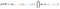Equation 2 . Note: (x1, …, xn) is the input sequence, (y1, …, ym) is the output sequence, and v the fixed-dimensional output of the encoder.

The model computes the conditional probability by first getting the fixed- dimensional representation v of the input sequence (x1, …, xn) given by the last hidden state of the LSTM and then computing the probability of (y1, …, ym) with a standard LSTM-LM formulation where the initial hidden state, v, is the representation of (x1, …, xn).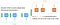Figure 1 (inspired by blog ). A more detailed view (described above).

The authors test their method on 2 types of experiments. Both experiments involve the English to French translation task using the WMT-14 dataset.

Experiment 1: They use the model to directly translate the input sequence without using a reference statistical machine translation (SMT) system. The Sequence-to-Sequence model outperforms the SMT baseline and was by far the best result achieved by direct translation with DNNs at the time.

Experiment 2: They use the model to rescore the 1000-best lists of an SMT baseline. The Sequence-to-Sequence model improves the baseline by 3.2 points and achieves very close to state-of-the-art results.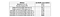Table 1 (from paper ). Results of experiment (1). Note: baseline system refers to SMT system.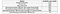Table 2 (from paper ). Results of experiment (2). Note: state-of-the-art refers to “Edinburgh’s Phrase-based Machine Translation Systems for WMT-14”.
1. Two LSTMS: Using two LSTMs enables simultaneous training on multiple language pairs.
2. Deep LSTMs: Deep LSTMs greatly outperform shallow LSTMs. Specifically, each additional layer reduced model perplexity by nearly 10%. The authors decide on 4 layers for each LSTM and 1000 cells at each layer.
3. Reversing input sequence: Arguably the most important technical contribution of this paper is the degree of improvement achieved by simply reversing the order of the input sequence. The authors suggest that this is caused by the introduction of many short-term dependencies to the dataset. Reversing the source order greatly reduces the minimal time lag while leaving the average distance between corresponding words in source and target unchanged. This makes it easier for back-propagation to establish communication between the source and the target, leading to vastly improved performance. This is also the reason why this particular method works well for translation of very long sentences.
4. Qualities of representations: The representation vectors are sensitive to the order of the words and are relatively insensitive to the replacement of active voice with a passive voice.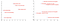Figure 2 (from paper ). 2-dimensional PCA projection of LSTM hidden states. Note that the visualization on the left demonstrates sensitivity to word order, and that on the right demonstrates relative invariance to the replacement of active voice with passive voice.

It is now known that using a single fixed-dimensional vector (as in the standard Sequence to Sequence model) to represent the input sequence leads to an information bottleneck. The attention mechanism helps to overcome this by allowing for a set of hidden representations that scales with the size of the source. Structured attention further extends this attention formulation by allowing the model to capture more complex structural biases.

# Structured Attention Networks

This paper, published in 2017, proposes an end-to-end approach to sequential learning with structured attention layers in place of simple attention. These networks allow for extending attention beyond standard soft-selection to graphical models like linear-chain conditional random fields and graph-based parsing models. Previously, graphical models like those proposed in this paper had mainly been incorporated into the last layer of the network, whereas this paper suggests that structured attention can be used in internal layers while still retaining the end-to-end trainable property.

The standard soft-selection attention procedure is a mechanism for computing the context vector. In place of a fixed context vector for decoding purposes, now there is a context vector that is conditioned on a given query variable (i.e. decoder hidden state) and is essentially a weighted sum over the memory bank (i.e. input sequence). To express this more rigorously, there is a set of inputs comprising a memory bank (x1, …, xn), a query variable q, and a random variable z that performs memory selection. P(z|x, q) is considered the attention distribution and the context vector is then ∑ P(z=i|x, q)xi, for i from 1 to n. See figure 3 below (left) for an illustration of this mechanism.

Structured attention replaces the attention distribution P(z|x, q) with a distribution over a combinatorial set of structures, where z is no longer a single variable but instead a vector of discrete latent variables z = [z1, …, zm]. The differences can be illustrated via example. Instead of soft-selecting a single input, suppose now we want to model the selection of contiguous subsequences. Let z = [z1, …, zn], where zi is either 0 or 1 (representing the selection of a given input element in the subsequence). The context vector is then ∑ P(zi=1|x, q)xi, for i from 1 to n. This procedure is fundamentally different from standard selection in 2 key ways: (i) it allows for multiple inputs or no inputs to be selected for a given query variables; (ii) it allows for the modeling of structural dependencies across zi’s. See figure 3 below (right) for an illustration of this mechanism.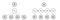Figure 3 (from paper). A standard soft-selection attention network (left) and a linear-chain structured attention model for segmentation (right).

The paper focuses on two main classes of structured attention.

1. Linear-chain conditional random field (CRF): This class is illustrated in the above example, in figure 7. Specifically, it is a statistical modeling method that implements sequential dependencies in predictions; in the paper, a linear-chain CRF is used to model the distribution over z.
2. Graph-based dependency parsing: This popular method for NLP tasks involves a dependency tree, which enforces certain parsing constraints (e.g. exactly one head word per word in the source sentence) and encourages the system to make soft-selection based on learned, recursive syntactic dependencies that are common in many languages. The mathematical formulation (i.e. the analog to the subsequence selection formulation in the previous section of this blog) can be found in Section 3.2 of the paper.

The authors design and test structured attention models in 4 experiments.

Experiment 1: Tree Transduction (using graph-based dependency parsing): This task is to convert a mathematical formula in prefix notation to one in infix notation and the authors’ innovation is the introduction of structured attention (dependency tree parsing) layers in an encoder-decoder LSTM model. The authors’ model significantly outperforms (>10% improvement in average length to failure) models that incorporate simple attention because the structured attention network partially reconstructs the arithmetic tree.Figure 4 (from paper). Mathematical formula in prefix notation → infix notation.

Experiment 2: Neural Machine Translation (using linear-chain CRF): This task is to translate Japanese characters to English words and the authors’ innovation is the introduction of structured attention (two-state CRF) layers in an encoder-decoder LSTM model. Since the simple model tends to focus a lot of attention on a single character and the new model is able to distribute attention across contiguous subsequences, structured attention provides improvements in performance (1–1.5 increase in BLEU score).

Experiment 3: Question Answering (using linear-chain CRF): This task is to answer a question based on a set of sentences/facts and the authors’ innovation is the introduction of structured attention (linear-chain CRF) over multiple facts. While existing approaches greedily attend to different facts, the authors use structured attention to perform non-greedy inference. For some tasks, the new model exhibits significant improvement, but for most tasks, higher sequence selection accuracy does not effect performance much because it is sufficient to select only the first or last fact to determine the answer.

Experiment 4: Natural Language Inference (using graph-based dependency parsing): This task is to predict the relationship (entailment, contradiction, neutral) between two sentences, and uses a model similar to that used in tree transduction. The structured attention model demonstrates improvements over models with simple attention (.6% in accuracy) and moreover, the syntactic attention layer learns a plausible dependency structure.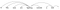Figure 5 (from paper). Hard parse for an example sentence, recovered by running the Viterbi algorithm on the syntactic attention layer.
1. Structured attention in internal layers: The authors’ approach is novel in terms of their use of structured attention in internal layers. As demonstrated, not only does this modification provide improvements in performance, but it also leads to learned representations that more accurately capture complex structural biases.
2. End-to-end training: The authors emphasize that the models proposed can be trained end-to-end. For example, in the linear CRF example, the attention distribution can be calculated efficiently using the forward-backward algorithm. Such a technique, like many other inference techniques, yields a context vector that is fully differentiable. Consequently, a structured attention model can be trained end-to-end.

# Observations and Insights:

1. It was a novel approach at the time to reverse the input sequence and it’s interesting to note that simply using the reversal (rather than both directions) allows the LSTM to predict longer sequences.
2. From a performance perspective, the authors found that this model is easy to train, which is very important in practice.
3. As mentioned, the limitations of this architecture include the fixed size context vector, which was later alleviated using attention and further extended using structured attention.
1. The formulation of structured attention is general enough to provide many different ways to explore latent structures and structured predictions; the paper only mentions 2 large classes but theoretically, there are endless possibilities for defining structured selection.
2. Although the experiments did not show leaps and bounds of improvement over simple attention in every instance, the instances in which the underlying structure is important show drastic improvement. The possibilities of modeling different structures makes this a very exciting field!

# Citations:

 Sutskever, I., Vinyals, O., & Le, Q. (2014, December 14). Sequence to Sequence Learning with Neural Networks. Retrieved November 29, 2020, from https://arxiv.org/abs/1409.3215

 Kim, Y., Denton, C., Hoang, L., & Rush, A. (2017, February 16). Structured Attention Networks. Retrieved November 29, 2020, from https://arxiv.org/abs/1702.00887

 Bahdanau, D., Cho, K., & Bengio, Y. (2016, May 19). Neural Machine Translation by Jointly Learning to Align and Translate. Retrieved November 30, 2020, from https://arxiv.org/abs/1409.0473

 Harvard NLP. (n.d.). Retrieved November 29, 2020, from https://nlp.seas.harvard.edu/

 Alammar, J. (n.d.). Visualizing A Neural Machine Translation Model (Mechanics of Seq2seq Models With Attention). Retrieved November 29, 2020, from http://jalammar.github.io/visualizing-neural-machine-translation-mechanics-of-seq2seq-models-with-attention/